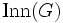# FZ-group

This article defines a group property: a property that can be evaluated to true/false for any given group, invariant under isomorphism
View a complete list of group properties
VIEW RELATED: Group property implications | Group property non-implications |Group metaproperty satisfactions | Group metaproperty dissatisfactions | Group property satisfactions | Group property dissatisfactions
This article defines a term that has been used or referenced in a journal article or standard publication, but may not be generally accepted by the mathematical community as a standard term.[SHOW MORE]

## Definition

### Equivalent definitions in tabular format

No. Shorthand A group is termed a FZ-group if ... A group$G$ is termed a FZ-group if ...
1 center has finite index its center is a subgroup of finite index in it. the center$Z(G)$ is a subgroup of finite index, i.e.,$G/Z(G)$ is finite.
2 inner automorphism group is finite its inner automorphism group is a finite group. the group$\operatorname{Inn}(G)$ of inner automorphisms (conjugations by elements of$G$) is finite.
3 inner automorphism group and derived subgroup both finite both its inner automorphism group and its derived subgroup are finite groups. the subgroups$\operatorname{Inn}(G)$ and$[G,G]$ are both finite groups.
4 isoclinic to a finite group it is isoclinic to a finite group. there is a finite group$K$ such that$G$ and$K$ are isoclinic groups.

## Relation with other properties

### Stronger properties

Property Meaning Proof of implication Proof of implication Proof of strictness (reverse implication failure) Intermediate notions
finite group the whole group is finite any infinite abelian group gives a counterexample. Finitely generated FZ-group|FULL LIST, MORE INFO
abelian group any two elements commute any finite non-abelian group gives a counterexample. |FULL LIST, MORE INFO

### Weaker properties

Property Meaning Proof of implication Proof of implication Proof of strictness (reverse implication failure) Intermediate notions
Stronger than:group with finite derived subgroup finitely many commutators, or equivalently, the derived subgroup is finite. FZ implies finite derived subgroup finite derived subgroup not implies FZ |FULL LIST, MORE INFO
group satisfying generalized subnormal join property an arbitrary join of subnormal subgroups is subnormal. |FULL LIST, MORE INFO
group satisfying subnormal join property a join of finitely many subnormal subgroups is subnormal. |FULL LIST, MORE INFO
FC-group every conjugacy class is finite. (via finite derived subgroup) Group with finite derived subgroup|FULL LIST, MORE INFO
BFC-group there is a common finite bound on the sizes of all conjugacy classes. Group with finite derived subgroup|FULL LIST, MORE INFO
virtually abelian group has an abelian subgroup of finite index. |FULL LIST, MORE INFO

## Metaproperties

### Direct products

This group property is finite direct product-closed, viz the direct product of a finite collection of groups each having the property, also has the property
View other finite direct product-closed group properties

A direct product of finitely many FZ-groups is an FZ-group. This follows from the fact that the center is a direct product-preserved subgroup-defining function, that is, the center of a direct product of groups is the direct product of their centers.